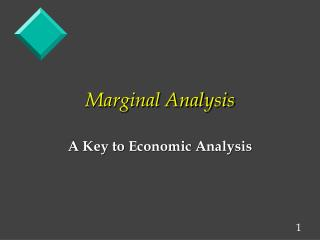DownloadDownload PresentationMarginal Analysis

# Marginal Analysis

Download Presentation## Marginal Analysis

- - - - - - - - - - - - - - - - - - - - - - - - - - - E N D - - - - - - - - - - - - - - - - - - - - - - - - - - -
##### Presentation Transcript

1. Marginal Analysis A Key to Economic Analysis

2. Marginal Analysis Marginal analysis is used to assist people in allocating their scarce resources to maximize the benefit of the output produced. Simply getting the most value for the resources used.

3. Marginal Analysis Marginal analysis: The analysis of the benefits and costs of the marginal unit of a good or input. (Marginal = the next unit)

4. Marginal Analysis A technique widely used in business decision-making and ties together much of economic thought. In any situation, people want to maximize net benefits: Net Benefits = Total Benefits - Total Costs

5. The Control Variable To do marginal analysis, we can change a variable, such as the: • quantity of a good you buy, • the quantity of output you produce, or • the quantity of an input you use. This variable is called the control variable .

6. The Control Variable Marginal analysis focuses upon whether the control variable should be increased by one more unit or not.

7. Key Procedure for Using Marginal Analysis 1. Identify the control variable (cv). 2. Determine what the increase in total benefits would be if one more unit of the control variable were added. This is the marginal benefit of the added unit.

8. Key Procedure for Using Marginal Analysis 3. Determine what the increase in total cost would be if one more unit of the control variable were added. This is the marginal cost of the added unit.

9. Key Procedure for Using Marginal Analysis 4. If the unit's marginal benefit exceeds (or equals) its marginal cost, it should be added.

10. Key Procedure for Using Marginal Analysis Remember to look only at the changes in total benefits and total costs. If a particular cost or benefit does not change, IGNORE IT !

11. Why Does This Work? Because: Marginal Benefit = Increase in Total Benefits per unit of control variable TR / Qcv = MR where cv = control variable

12. Why Does This Work? Marginal Cost = Increase in Total Costs per unit of control variable TC / Qcv = MC

13. Why Does This Work? So: Change in Net Benefits = Marginal Benefit - Marginal Cost

14. Why Does This Work? When marginal benefits exceed marginal cost, net benefits go up. So the marginal unit of the control variable should be added.

15. Example: Should a firm produce more ? A firm's net benefit of being in business is PROFIT. The following equation calculates profit: PROFIT = TOTAL REVENUE - TOTAL COST

16. Example: Should a firm produce more ? Where: TR = (Poutput X Qoutput) n TC =  (Pinputi X Qinputi) i=1 Assume the firm's control variable is the output it produces.

17. Problem: International Widget is producing fifty widgets at a total cost of \$50,000 and is selling them for \$1,200 each for a total revenue of \$60,000. If it produces a fifty-first widget, its total revenue will be \$61,200 and its total cost will be \$51,500.

18. Problem: Should the firm produce the fifty-first widget?

19. Answer: NO The fifty-first widget's marginal benefit is \$1,200 (\$61,200 - \$60,000) / 1 This is the change in total revenue from producing one additional widget and is called marginal revenue.

20. Answer: The firm's marginal cost is \$1,500 (\$51,500 - \$50,000) / 1 This is the change in total cost from producing one additional widget. This extra widget should NOT be produced because it does not add to profit:

21. Answer: Change in Net Revenue (Benefit) = Marginal Revenue - Marginal Cost - \$300 = \$1,200 - \$1,500

22. Qcv Qwidgets TR TR TC TC 50 60,000 50,000 11,200 1,500 51 61,200 51,500 MR = TR / Qcv= \$1,200 / 1 = \$1,200 MC = TC / Qcv=\$1,500 / 1 = \$1,500

23. A Question: What is the minimum price consumers would have to pay to get a 51st Widget produced? • Consumers would have to pay at least \$1,500 for the extra widget to get the producer to increase production.

24. Summary • Marginal analysis forms the basis of economic reasoning. • To aid in decision-making, marginal analysis looks at the effects of a small change in the control variable.

25. Summary • Each small change produces some good (its marginal benefit) and some bad (its marginal cost). • As long as there is more "good" than "bad", the control variable should be increased (since net benefits will then be increased).

26. Practical Exercise: Turn to the class exercise in your Notebooks. Please complete the class exercise.